## General Chemistry

Learn the toughest concepts covered in Chemistry with step-by-step video tutorials and practice problems by world-class tutors

16. Chemical Equilibrium

# ICE Chart

In order to calculate the equilibrium concentrations of compounds we need to use an ICE Chart.

Calculating Equilibrium Concentrations
1
concept

## Understanding an ICE Chart2m
Play a video:
Hey guys in this new video, we're gonna do something incredibly important calculating equilibrium concentrations. Now, up to this point, I've been giving you questions in which our reaction is at equilibrium and the numbers I'm giving you are equilibrium amounts. Now, we're gonna be tasked with figuring out what those equilibrium amounts when I give you just initial concentrations. Now remember initial concentrations and equilibrium concentrations are totally different. And we're gonna say sometimes we'll be asked to calculate concentration of equilibrium. After being given initial concentrations, we're gonna say in order to do this, we're gonna have to use our favorite friend the ice chart and remember what does I stand for? I stands for initial change equilibrium. And we're gonna learn how to use an ice chart. And when do we use an ice chart? And what we should realize here is that ice charts are only allowed to have two types of units. We're gonna say they're used to having atmospheres or molar as the units. And remember why those two units because atmospheres are connected to K P and molar is connected to K C. We're still gonna be dealing with our equilibrium constants because they go hand in hand with our ice charts. Now, when do we use an ice chart? You only ask yourself one question or really you say one thing you're gonna say any time we have more than one compound in our balanced equation without an equilibrium concentration, then we have to use an ice chart. So before we start this next question, what do I mean by this? In the next question, we have three compounds within our balanced equation. Let's say I gave you the equilibrium amounts for two of them, we'd only be missing one person at equilibrium. In that case, we wouldn't need to do an ice chart. But let's say I gave you only one person at equilibrium. Technically, we'd have to do an ice chart in order to organize our work to see what the correct answer would be. So again, if you're missing more than one compound at equilibrium, you should use an ice chart. And if you don't have anyone at equilibrium, then you should definitely use an ice chart.

An ICE Chart should be used when we are missing more than one equilibrium amount for compounds in our balanced equation.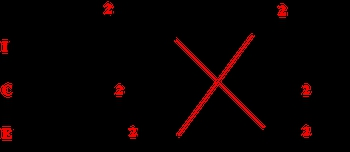Since our equilibrium constant K is involved in an ICE Chart then we must continue to ignore solids and liquids.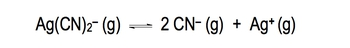2
example

## We have a solution where Ag(CN)2 –(g), CN – (g), and Ag+ (g) have an equilibrium constant, K, equal to 1.8 x 10-19. If the equilibrium concentrations of Ag(CN)2 – and CN – are 0.030 and 0.10 respectively, what is the equilibrium concentration of Ag+3m
Play a video:
So let's take a look at the first two examples and see how we approach these types of questions. We say here when we have a solution where A G C N two minus gas, C N minus gas and A G positive gas and they have an equilibrium constant K equal to 1.8 times 10 to the negative 19. If the equilibrium concentrations of AGCN 2 - and CN are these numbers respectively. What is the equilibrium concentration of A G positive? So we ask ourselves, do we use a nice chart or not here? I give you the equilibrium amounts of this compound which is 0.30 and this compound which is 0.10, you're missing only one equilibrium amount. If you're missing only one equilibrium amount, then you don't have to do an ice chart. So we're simply gonna say in this case that K equals products over reactants. Yeah, we say that products here would be C N minus squared times A G positive. And since we're gonna need some room guys, I'm gonna remove myself from the image. So we have more room to work with divided by AGCN 2 negative. So what we're gonna do now is we're gonna plug in the numbers that we know. We know what K is. It's 1.8 times 10 to the negative 19 C N negative we said was .10 and it's gonna be squared A G positive is what we're looking for. So we're gonna have it as a variable divided by A G C N two minus which is 20.30. OK. So this question becomes fairly easy. All we have to do now is isolate our A G positive. So what we're gonna do first is multiply both sides by 0.30, these two multiply together to give us a new answer of 5.4 times 10 to the -21. And that equals .10 squared times A G positive. We just want a G positive. So we're gonna divide it out the 0.10 squared. So our final answer at the end for silver ion will be 5.4 times 10 to the negative 19 molar. So again, why didn't we have to use an ice chart? We didn't have to use an ice chart because we're missing only one person at equilibrium when you're missing no one at equilibrium or you're missing only one variable at equilibrium. Then you don't need to do an ice chart. We only do an ice chart when more than when, more than one concentration is missing at equilibrium.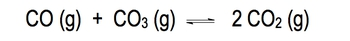3
example

## We place 2.5 mol of CO and 2.5 mol of CO3 in a 10.0 L flask and let the system come to equilibrium7m
Play a video:
4
Problem

For the reaction:N2 (g) + 2 O2 (g) ⇌ 2 NO2 (g), Kc = 8.3 x 10 -10 at 25°C. What is the concentration of N2 gas at equilibrium when the concentration of NO2 is twice the concentration of O2 gas?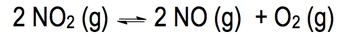5
example

## When 0.600 atm of NO­­2 was allowed to come to equilibrium the total pressure was 0.875 atm5m
Play a video:
Hey, guys, In this brand new video, we're gonna continue with our conversation on calculating equilibrium Concentrations. So let's take a look at this particular question we're gonna say when 0. atmospheres of n 02 was allowed to come to equilibrium, the total pressure was 20.875 atmospheres calculate the KP of the reaction. Now all I'm giving you is 0.600 atmospheres of Eno to I'm saying I'm allowed me to come to equilibrium. What that tells me is this amount is not my equilibrium amount. It's rather my initial amount. So this is my initial pressure. So all we say to ourselves is Do we have to use a nice chart? And the answer here is Yes, because we're gonna have this missing equilibrium amount. This is missing equilibrium amount, and this is missing an equilibrium amount. Any time. More than one of my compounds in my balanced equation is missing an equilibrium amount. I have to do a nice chart. This number here I'm giving it time to get to equilibrium, which means it's my initial pressure. So we're gonna say I is initial. See, it's change. E is equilibrium. So we're gonna say Initially, we're starting out with 0.600 atmospheres. Remember Atmospheres Goals with KP. I don't tell us anything about N. O or 02 so they're initially at zero. Remember the important phrase we've been saying? We're losing reactant to make products. So this is going to be minus two X because of the two and it's minus because it's reacted. Plus two x the two there. That's why it's plus two x plus x Bring down everything. 0.600 minus two X plus two X plus X. Now what we should realize here is they're telling me what the total pressure is now this goes back to Henry's law or actually not Henry's Law. But more importantly, Dalton's law Dalton's Law says that the total pressure that we experience is equal to the pressure of all of the gas is added up. So my total pressure is equal to the pressure of n 02 plus the pressure of Eno plus the pressure of +02 and at equilibrium. That's my equilibrium. Total pressure. So what we're gonna do here is say, at equilibrium. Each of these gasses is equal Thio Each of these equations. So at equilibrium are total pressures. 0.875 atmospheres at equilibrium N 02 is 20.600 minus two x at equilibrium. Eno is two x at equilibrium. 02 was just X. We're going to say that this negative to in this positive to basically cancel each other out. So our equation becomes 0. atmospheres equals 0.600 plus X. We need to isolate X here, so we're gonna subtract 0.600 0.600. So x here equals 0.275 atmospheres. Remember, this is Dalton's law. The total pressure we experience is the pressure from all of the gas is added up together. And this is this an equilibrium, total pressure. We use the equilibrium equations for each of the compounds we just isolated. What? Exes. So all we need to do is take this X answer, plug it in here, plug it in here, plug it in here and we'll know each of them at equilibrium. And if we know each of them at equilibrium, then it's those equilibrium amounts that we plug into KP. Remember, KP is just products overreacting. So it's Enel squared times out to divided by N 02 squared because the two on the two here. So when we plug in the X in for N we get 0.50 atmospheres when we plug it in for N o at equilibrium and ah equals two x plug in the X. And so we just found. So that's a no. And then 02 is just equal to exit equilibrium. So with that same exact number now take all those numbers and plug it in. Okay? And when you do all that, you'll get your KP equal to 33. Now, to do this question, you had to have a knowledge off Dalton's law. Remember that deals with gasses and then apply that concept to this new idea of a nice chart. So it's kind of taking a little bit of old information, combining with some new information in order to obtain R K P value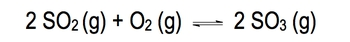6
example

## An important reaction in the formation of acid rain listed below.2 SO2 (g)   +  O2 (g)  ⇌  2 SO3 (g)Initially, 0.023 M SO2 and 0.015 M O2 are mixed and allowed to react in an evacuated flask at 340 oC6m
Play a video:
So the next question says an important reaction in the formation of acid rain is to eso to gas is combined with 102 gas to give us to eso three gas Here we're saying initially, we have 0.23 Moeller s 02 and 0.15 Mueller 02 They're mixed together and allowed to react in an evacuated flask at 340 degrees Celsius. When an equilibrium is established, the equilibrium amount of one of them s 03 was found to be 30.199 Moeller, calculate the equilibrium constant K C for the reaction at 340 degrees Celsius. So we say to ourselves, Do we need to do a nice chart? Well, we know initially this is how much we have of our react INTs equilibrium is reached, but we only know the equilibrium of one compound. So we're still missing the equilibrium from eso to and for Anytime we're missing more than one compound and equilibrium, we have to do a nice shirt. So in this case, we're gonna do a nice chart. Now I'm gonna remove myself from the image guy so that we can work this problem out with more space. So let's take a look. We know we have to do a nice chart, so it's gonna be to s 02 plus o two. Gas gives me to s 03 gas initial change equilibrium. So now what we're gonna do here is we're gonna We're gonna plug in what we know initially. So initially, we know the amount for s 02 is 20.23 Moeller and we know what the initial amount of 02 is. It's gonna be point 015 Moeller. I don't tell you anything about s 03 So initially it's going to be equal to zero. Then we're gonna say, Remember the important phrase we lose react INTs to make products. This helps us figure out how we exactly we put in the change. So it's gonna be minus two x because of the two minus X plus two acts because of the two bring down everything to 3 minus two x 0.15 minus x plus two x Hopefully you guys were able to fill out your ice chart in the same way, as I did next, we're gonna say the important thing here is we know what s 03 is at equilibrium at equilibrium. Eso three equals two X. But the important thing here that we should realizes that I tell us another piece of information I actually tell us what s 03 is at equilibrium. At equilibrium, it equals 30.19 So that means two X also equals 20. Because we know this, we can isolate our x variable. So we're gonna divide. Both sides were gonna divide this by two and this by to toe isolate our x variable. So we're gonna stay here. X equals point 0009 Mueller. And now, because we know what X is, we can take that X answer, and we can plug it in for the other compounds. So we plug it in for this x and in this X So we're gonna say eso to equilibrium equals 0.23 minus two x. We just solve what? The exes. So when we work that out, we'll find out what our answer is for. Okay. Here. No equal 0.2101 Now we're gonna do the same thing for 02 At equilibrium 02 equals 20.15 minus X plug in what we know for X. So it equals 0. Okay, now we know everyone in equilibrium. So now we're able to find Casey. K C equals products, overreact INTs. So all we do now is we plug in their equilibrium amount that we just calculated. So we know SL three equilibrium is 30.199 squared S 02 is 20.2101 squared. And so too is just point 4005 Plug all that in and we'll get our answer. Okay. When we do that, our Casey is 0.641 as our answer. Hopefully, you guys were able to see the connections that we needed here. We're only given one person at equilibrium. So technically, we should have done a nice chart. The great thing is because we knew one person that equilibrium, we could isolate our X. Once we isolated Rx, we take that answer and plug it into each one of the compounds. Add the equilibrium line and doing that helped us find out what Casey is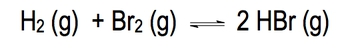7
example

## If Kc is 32.7 at 300oC for the reaction below7m
Play a video:
8
Problem

At a given temperature the gas phase reaction:N2 (g) + O2 (g) ⇌ 2 NO (g) has an equilibrium constant of 4.00 x 10 -15. What will be the concentration of NO at equilibrium if 2.00 moles of nitrogen and 6.00 moles oxygen are allowed to come to equilibrium in a 2.0 L flask.

9
example